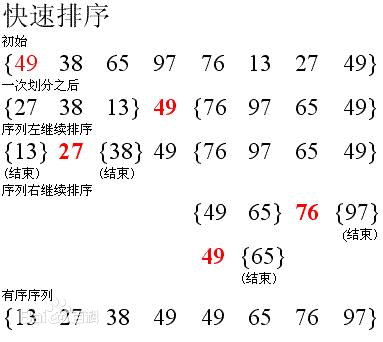# PHP 快速排序算法详解PHP实现

/*
快速排序
*/

function quickSort(\$array)
{
if(!isset(\$array))
return \$array;
\$mid = \$array; //获取一个用于分割的关键字，一般是首个元素
\$leftArray = array();
\$rightArray = array();

foreach(\$array as \$v)
{
if(\$v > \$mid)
\$rightArray[] = \$v;  //把比\$mid大的数放到一个数组里
if(\$v < \$mid)
\$leftArray[] = \$v;   //把比\$mid小的数放到另一个数组里
}

\$leftArray = quickSort(\$leftArray); //把比较小的数组再一次进行分割
\$leftArray[] = \$mid;        //把分割的元素加到小的数组后面，不能忘了它哦

\$rightArray = quickSort(\$rightArray);  //把比较大的数组再一次进行分割
return array_merge(\$leftArray,\$rightArray);  //组合两个结果
}

\$a = array_rand(range(1,3000), 1500);  //甚至在冒泡算法超过1600个元素的时候会出现内存不足的提示，但这里为了测出两个之间的差别大小， 就设置成了1500，保证冒泡算法也能执行完毕。
shuffle(\$a);  //获取已经打乱了顺序的数组
\$t1 = microtime(true);
quickSort(\$a);  //快速排序
\$t2 = microtime(true);
echo ((\$t2-\$t1)*1000).'ms<br/>';

require('./maopao.php');  //这里引用的是我之前写的冒泡算法排序
\$t1 = microtime(true);
maoPao(\$a);   //冒泡
\$t2 = microtime(true);
echo ((\$t2-\$t1)*1000).'ms';

12.10880279541ms
772.64094352722ms